Parameter RecoveryNext: Results Up: Simulations Previous: Confidence Intervals

Parameter Recovery

At a fixed total observed emission line flux, the signal-to-noise (S/N) ratio in spatially-resolved spectra depends on the apparent size and Vsinof the target galaxies. In read-out and sky photon noise limited spectra, the rotation curve of small, slow rotating galaxies have higher S/N ratios because the emission line flux is spread over a smaller area on the CCD detector. The complex interplay between flux, intrinsic size, rotation velocity and redshift yielding the final S/N values can only be thoroughly modelled through simulations. ELFIT2D was tested on a set of simulations spanning a wide range of parameter values and hence, a wide range of S/N ratios to look for systematic biases and parameter covariances. A total of 342 rotation curve simulations were analyzed with ELFIT2D.

The simulated rotation curves were created using an IRAF/SPP task called GO2DBLT which produced simulated spectra of the [OII] doublet following the description of synthetic rotation curves given in section. Photon and read-out noise was added using the IRAF task MKNOISE in the ARTDATA package. Each simulated rotation curve was the sum of two 3600s exposures and was analyzed with ELFIT2D as if it were a real observed spectrum. Canonical parameter values were Vsin= 130 km/s, disk scale length of 2.0hkpc, [OII] ratio of 1.0, total [OII] flux of 1053 DU and background level of 0 DU. With these values, the S/N ratios in simulations were typically worse than the S/N ratios of observed spectra. For example, most target galaxies had disk scale length less than 2.0 hkpc (see Table), and only 4 out of 13 observed MOS spectra had total [OII] fluxes lower than 1053 DU. On the other hand, only 2 out of 9 SIS spectra had total [OII] fluxes higher than 1053 DU, so another set of simulations with total [OII] flux of 526 DU was generated. All observed SIS spectra had total [OII] fluxes higher than this lower flux limit.

Two main sets of simulations were created: one for SIS and one for MOS. Each set was further subdivided in low and high redshift subsets (z=0.25 and z=0.42), and each of these subsets comprised 5 sequences with each sequence exploring a range of values for a given parameter. The parameter values used in the five simulation sequences are given in Table. The independent variables in sequences 1, 2, 4 and 5 were disk scale length, Vsin, total [OII] flux and [OII] ratio respectively. Sequence 3 was made up of random combinations of parameter values. Note that the parameters for the sequences 1.3 and 2.3 are given in orderi.e. the first simulation had r= 2.5 hkpc, Vsin= 130 km/s and a [OII] ratio of 0.3, the second simulation had r= 1.0 hkpc, Vsin= 173 km/s and a [OII] ratio of 1.5, and so on. The total [OII] flux in all 1.x sequences was 1052 DU, and the total [OII] flux in the 2.x simulation sequences generated for SIS was 526 DU. A five-panel figure showing parameter variations measured by ELFIT2D as a function of input values of the independent variable was plotted for each sequence. The identification numbers labelling all these figures are given in Table. Tablegives Figure ID for sequences 1.1 through 1.5 and 2.1 through 2.5 generated for MOS and SIS at low (z=0.25) and high (z=0.42) redshifts.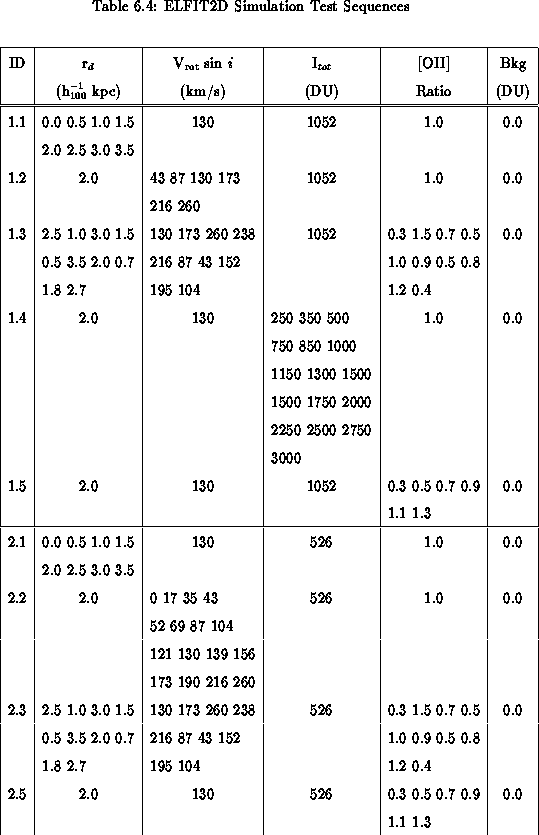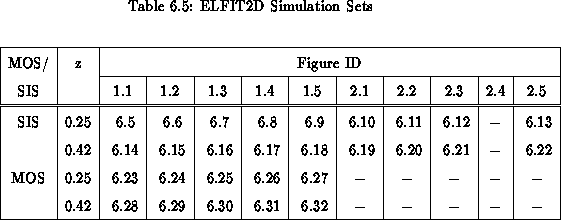For the simulated SIS rotation curves, ELFIT2D did a remarkable job at recovering the input parameter values even in the 2.x sequences which had very low total [OII] fluxes. For MOS, Figuresandshow that ELFIT2D does well at recovering parameter values (except for the [OII] ratio as expected from the spectral resolution of MOS), but worrisome biases are seen in other figures. In particular, Figureshows that, for MOS data, the scale lengths measured by ELFIT2D are consistently too low and the measured Vsin's are consistently too low for input Vsinvalues greater than 150 km/s. The total [OII] flux also appears to be systematically underestimated. These biases are what would be expected from spectra with S/N ratios that are too low. These biases are particularly worrisome because they affect two important variables. Disk scale length is used to determine whether [OII] is concentrated in the nuclei of galaxies, and Vsinis used to look for changes in luminosity. Figureshows very low S/N ratios introduce systematic errors which could be interpreted as anomalous [OII] kinematics and/or luminosity boosting.

One of the limitations of the body of simulations described so far is its set of canonical parameter values. Ideally, simulations should all be re-run for 22 different sets of canonical parameter values, one per observed spectrum. It would be the best way to test the performance of ELFIT2D on each spectrum. However, this would require generating close to 7500 simulations which could only be analyzed in a reasonable time with faster computers. Are the biases seen in the MOS simulations simply due to the fact that MOS simulations had lower S/N ratios than the observations? To answer this question, additional MOS simulations were generated. Sequence 2 was generated for each galaxy observed with MOS. The broad-band disk scale length taken from Tablewas used for the [OII] scale length. The total [OII] flux of the galaxy was taken to be its observed flux (Table). It is important to keep mind that the observed total [OII] flux could be an underestimate in some cases as suggested by Figure. The redshift of the simulations of a given galaxy was the redshift given in Tablefor that galaxy. Figurestoshow ELFIT2D measured disk scale length and Vsinas a function of input Vsinfor each galaxy observed with MOS. These figures are discussed galaxy by galaxy in Section.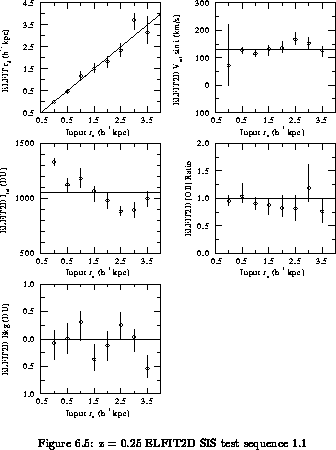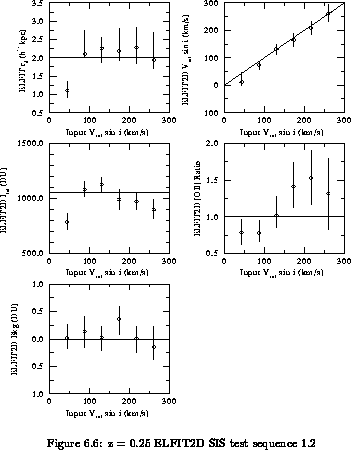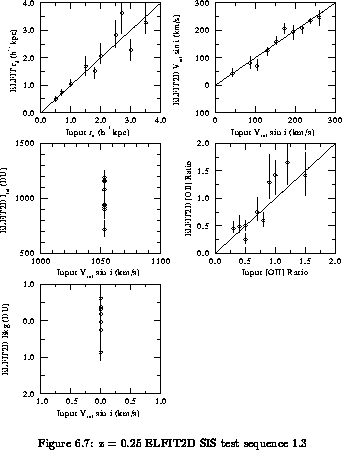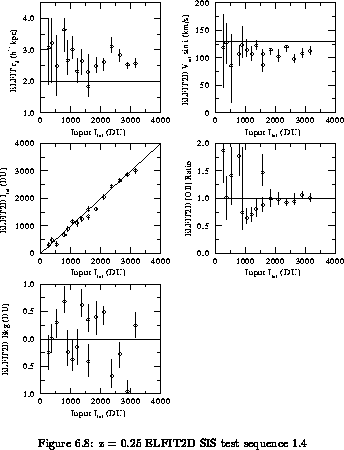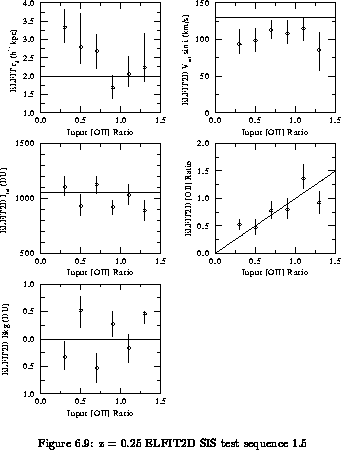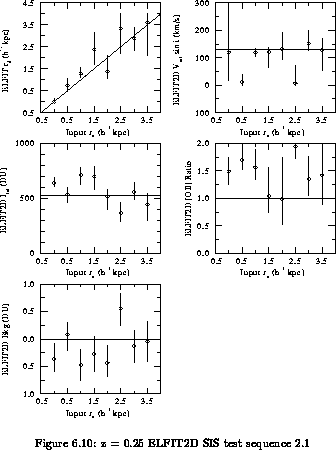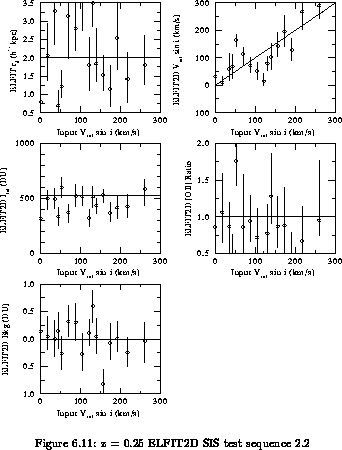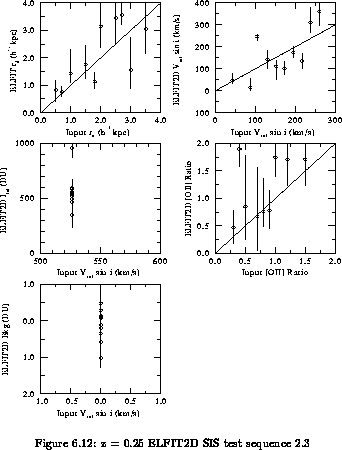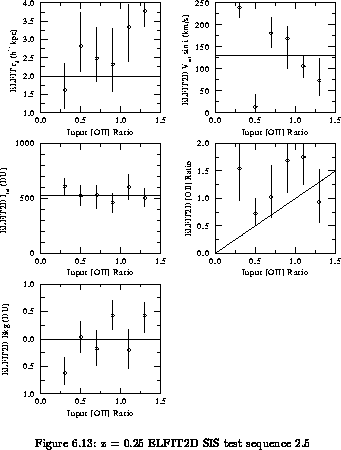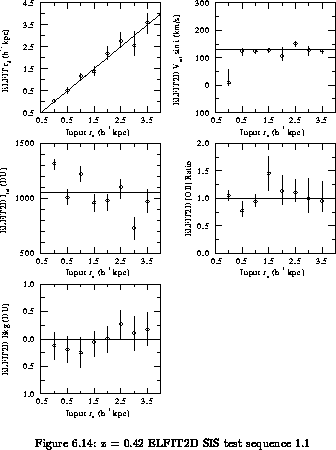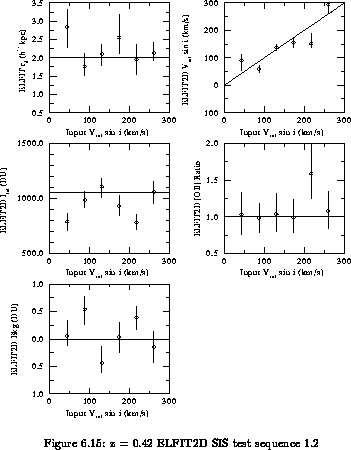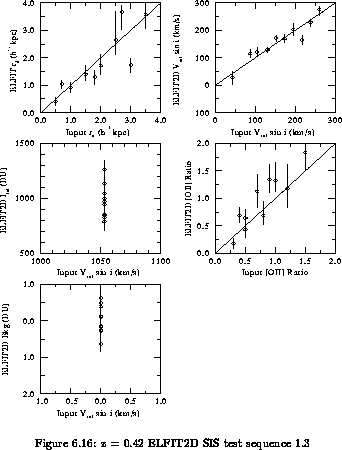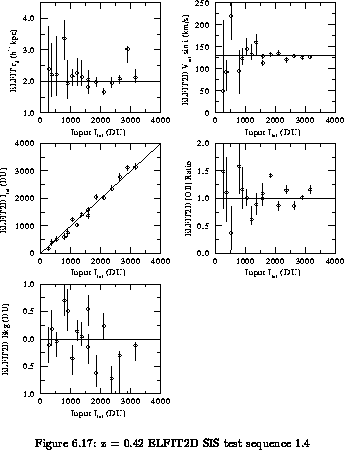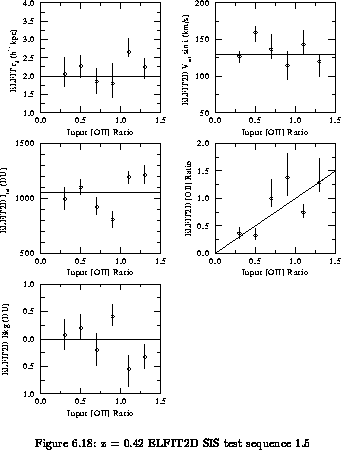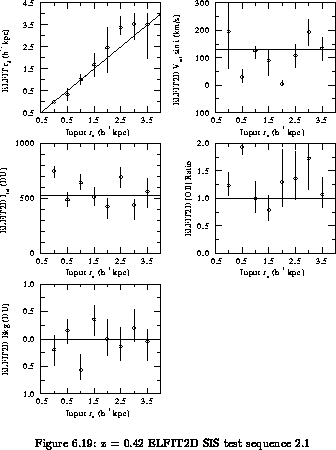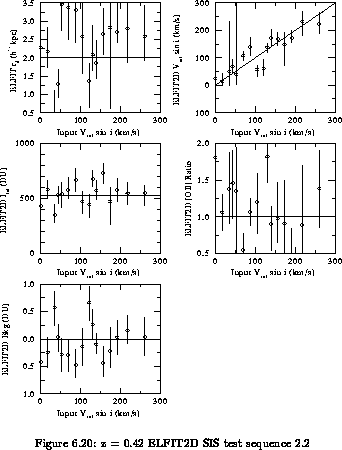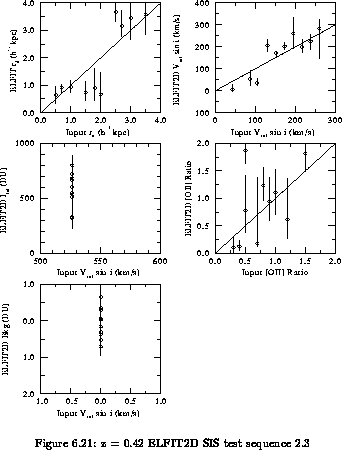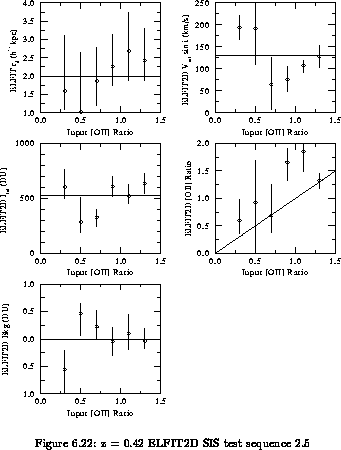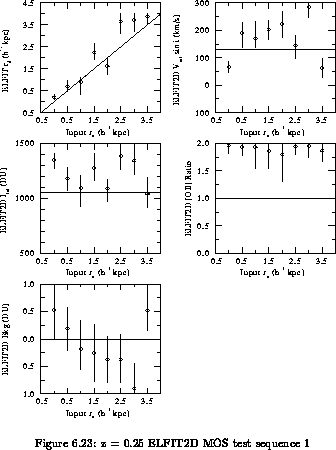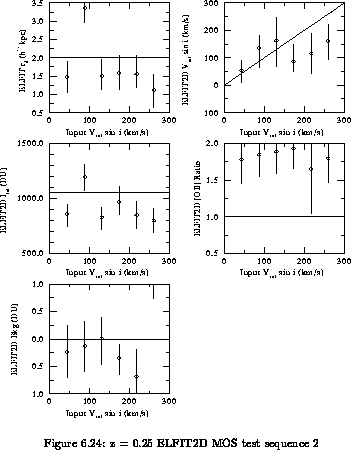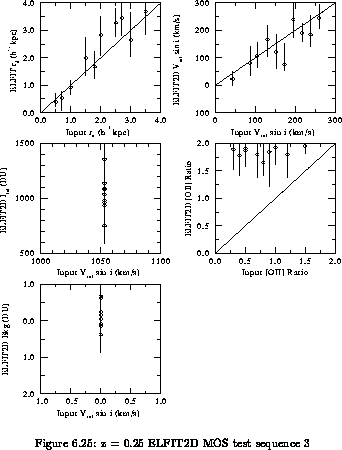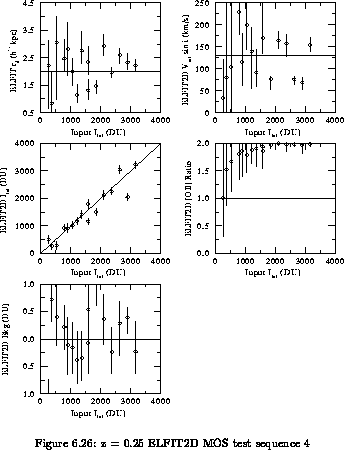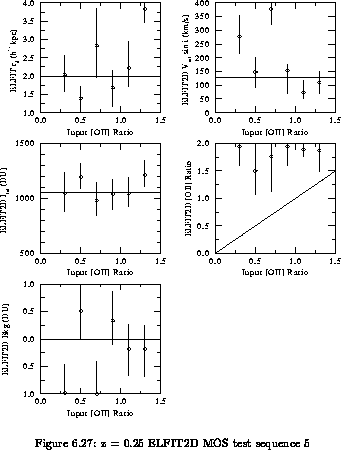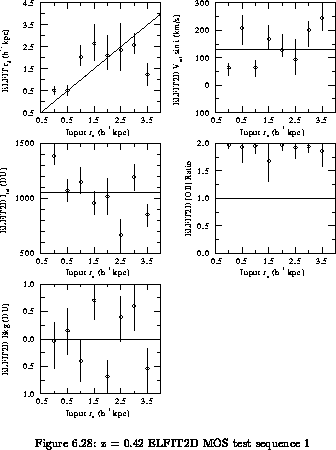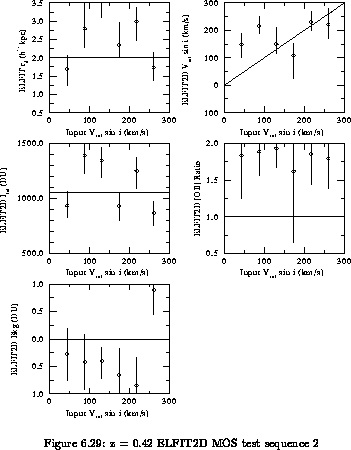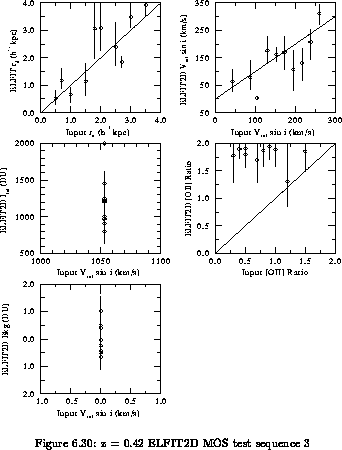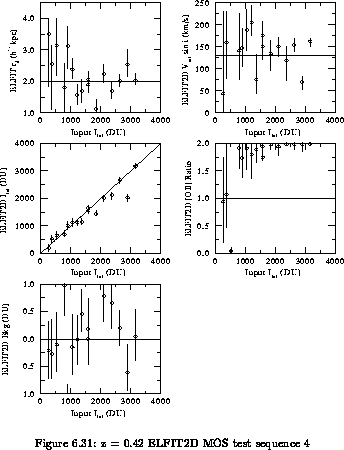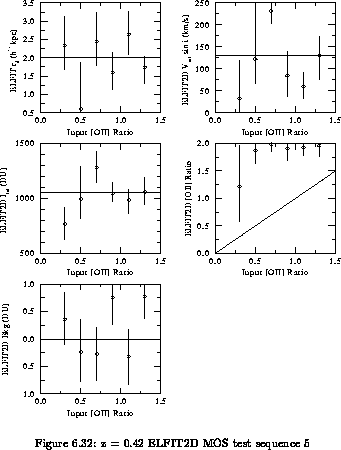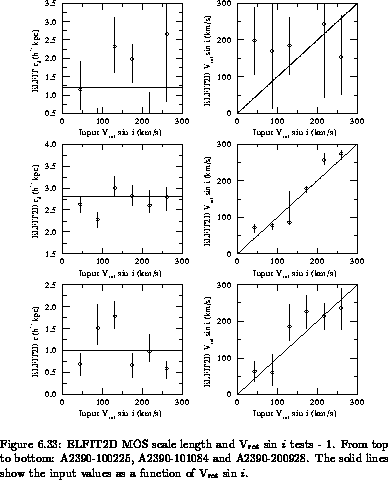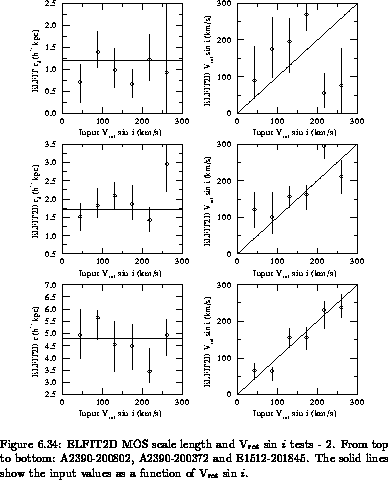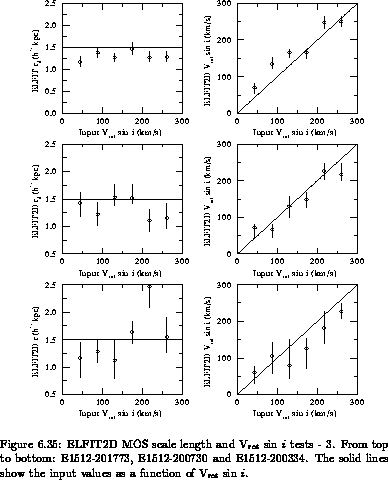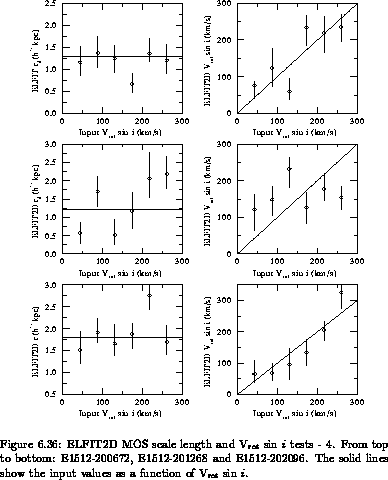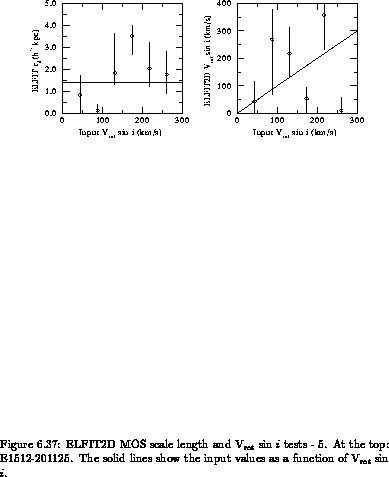Next: Results Up: Simulations Previous: Confidence Intervals

Luc Simard
Mon Sep 2 12:37:40 PDT 1996